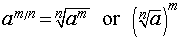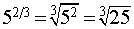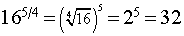index: click on a letter A B C D E F G H I J K L M N O P Q R S T U V W X Y Z A to Z index index: subject areas numbers & symbols sets, logic, proofs geometry algebra trigonometry advanced algebra & pre-calculus calculus advanced topics probability & statistics real world applications multimedia entrieswww.mathwords.com about mathwords website feedback

 Rational Exponents Fractional Exponents The use of rational numbers as exponents. A rational exponent represents both an integer exponent and an nth root. The root is found in the denominator (like a tree, the root is at the bottom), and the integer exponent is found in the numerator. Rule:Examples:See also